# Discrete programming

A branch of mathematics the subject of which is the study and solution of extremal problems on finite sets. Let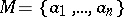and letbe a numerical function defined on the elements of. The task is to find an elementon which the absolute minimum (or the absolute maximum) ofonis obtained. Such problems are written down in an abbreviated form as follows: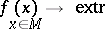Discrete programming deals only with the non-trivial problems in this class of problems, which meet the following additional conditions.

1) The numbermust be sufficiently large for the problem not to be solvable by direct inspections of the values of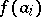manually or with the aid of an electronic computer. Thus, in the travelling salesman problem (which is a typical discrete programming problem), the number of possibilities to traversepoints is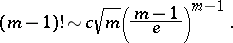In the problems of minimization of Boolean functions (cf. Boolean functions, minimization of; Boolean functions, metric theory of)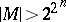.

2) The problem must not be regular. A problem is said to be regular if: a) for each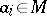there is a non-empty neighbourhoodand; b) a local extremum of, i.e. a point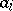such that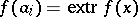,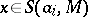, is defined by a simple algorithm; and c) a local extremum coincides with at least one global extremum.

Thus, discrete programming deals with problems involving, generally speaking, several local extrema. In typical cases the number of local extrema is very large. Thus, in problems of integer linear programming with Boolean variables, in which the objective function and the constraints depend on variables, the number of elements indoes not exceed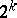, and the number of local extrema may be equal to. The degree of difficulty of solving discrete programming problems is determined by the presence of a large number of local extrema. At the time of writing (1988) there are no effective universal methods for solving discrete programming problems. Studies on the minimization of Boolean functions (which is a thoroughly studied model in discrete programming, cf. Algorithm, local) indicate that it is impossible to develop such methods. Sufficiently universal methods, such as branch-and-bound methods  and their various modifications, are methods based on implicit enumeration. They are effectively employed in solving specialized discrete programming problems. However, there is a wide class of problems for which any implicit enumeration method is only little less complex than explicit enumeration methods. Another source of mathematical difficulties in discrete programming problems is that the seton which the extremum ofis sought is often given in an implicit form. Thus, in problems of integer linear programming the setis defined as the collection of integer solutions of a system of linear inequalities. Ifis defined in this way or even in a more complex manner, not only the task of complete enumeration of, but even of specifying a single element ofbecomes a non-trivial problem. As a result, the main results in discrete programming were obtained in solving and studying narrower classes of problems. These include the transportation problem (cf. Transport problem), the travelling salesman problem and the multi-salesman problem, integer linear programming, scheduling problems (cf. Scheduling theory), extremal problems on graphs (cf. Graph theory), and problems on the minimal representation of Boolean functions and functions of-valued logic, etc.

Another trend in the theory of discrete extremal problems is the development of approximation methods, which are usually employed in solving large-size practical problems. In principle, there is no difference between these methods and the corresponding search methods for the extrema of continuous functions and functionals. In discrete programming the algorithms employed are analogues of algorithms of local optimization, descent, random search, etc. For a review of approximation methods of problem solving by discrete programming see .

The statistical approach to discrete programming problems is of both theoretical and of applied interest. Let a collection of problems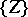be representable in the form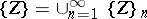, where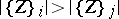if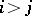andif. One says that the subsetcontains almost-all problems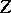ifThe following fact applies to various classes of discrete programming problems: There exists a collectionof almost-all problemsfor which it is possible to find the extremum or a good approximation to it in the class of simple algorithms; this collection is often effectively described. This was first noted in solving the problems of synthesis of optimal control systems, e.g. in the minimization of Boolean functions in the class of disjunctive normal forms (see Boolean functions, normal forms of, and also ). For instance, the problem of isolating the extremal conjunctions comprised in at least one minimal disjunctive normal form of a Boolean function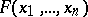is unsolvable in the class of local algorithms if, whereis the index of the local algorithm andis the size of the memory. At the same time the problem of isolating the elementary conjunctions forming part of at least one "almost-minimal" disjunctive normal form for almost-all Boolean functions is solvable in the class of local algorithms if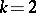,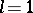. A similar considerable reduction of the labour involved in almost-all problems may be obtained for extremal problems on graphs, construction of optimal coverings, etc.

How to Cite This Entry:
Discrete programming. Encyclopedia of Mathematics. URL: http://encyclopediaofmath.org/index.php?title=Discrete_programming&oldid=24423
This article was adapted from an original article by Yu.I. Zhuravlev (originator), which appeared in Encyclopedia of Mathematics - ISBN 1402006098. See original article